# Javascript面试题和答案合集：哪些最常见？

2021年12月12日19:11:38 发表评论 298 次浏览

JavaScript 由 Brendan Eich 于 1995 年开发，是最流行的 Web 开发语言之一。

## 基本的Javascript面试题和答案合集：

### 1. javascript 中存在哪些不同的数据类型？

#### 原始类型

• 字符串 - 它代表一系列字符并用引号写入。字符串可以用单引号或双引号表示。

`````` var str =  "Vivek Singh Bisht" ; //使用双引号
var str2 =  'John Doe' ; //使用单引号``````
• Number - 它代表一个数字，可以写有或没有小数。

``````var x = 3; //without decimal
var y = 3.6; //with decimal``````
• BigInt - 此数据类型用于存储超出 Number 数据类型限制的数字。它可以存储大整数，并通过在整数文字上添加“n”来表示。

``var bigInteger = 234567890123456789012345678901234567890;``
• Boolean - 它代表一个逻辑实体，只能有两个值：true 或 false。布尔值通常用于条件测试。

``````var a = 2;
var b =  3;
var c =  2;
(a == b) // returns false
(a == c) //returns true``````
• Undefined - 当一个变量被声明但没有赋值时，它的值为 undefined 并且它的类型也是 undefined。

``````var x; // value of x is undefined
var y = undefined; // we can also set the value of a variable as undefined``````
• Null - 它代表不存在或无效的值。

``var z =  null;``
• Symbol - 它是 JavaScript 的 ES6 版本中引入的一种新数据类型。它用于存储匿名且唯一的值。

``var symbol1 = Symbol ( 'symbol' );``
• typeof 原始类型
``````typeof "John Doe" // Returns "string"
typeof 3.14 // Returns "number"
typeof true // Returns "boolean"
typeof 234567890123456789012345678901234567890n // Returns bigint
typeof undefined // Returns "undefined"
typeof null // Returns "object" (kind of a bug in JavaScript)
typeof Symbol('symbol') // Returns Symbol``````

``````// Collection of data in key-value pairs

var obj1 = {
x:  43,
y:  "Hello world!",
z: function(){
return this.x;
}
}

// Collection of data as an ordered list

var array1 = [5, "Hello", true, 4.1];   ``````

*注意- 重要的是要记住，任何不是原始数据类型的数据类型，在 javascript 中都是对象类型。

### 2. Javascript常见面试题有哪些：解释javascript的提升。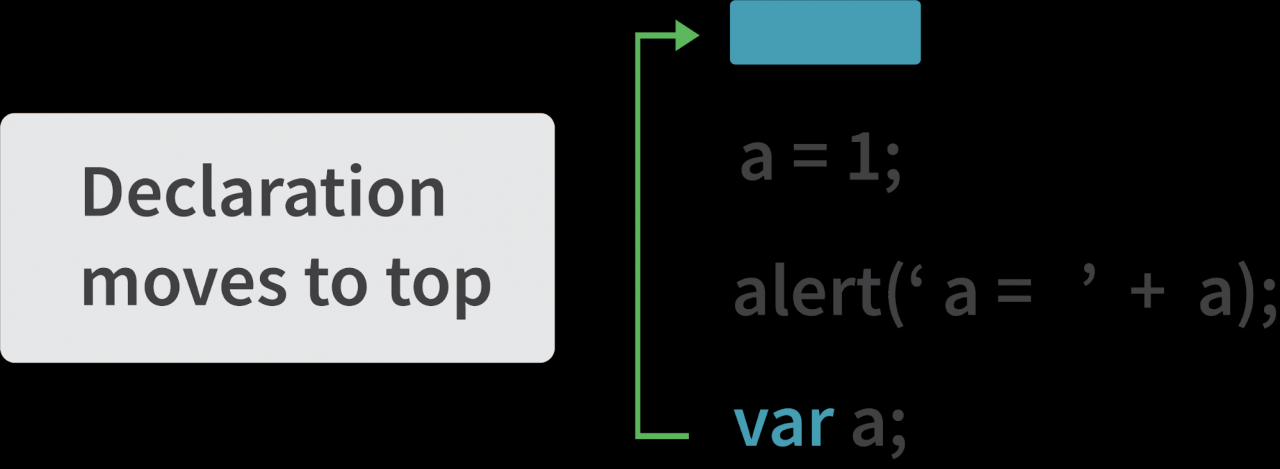Javascript面试题解析示例 1：

``````hoistedVariable = 3;
console.log(hoistedVariable); // outputs 3 even when the variable is declared after it is initialized
var hoistedVariable;``````

``````hoistedFunction();  // Outputs " Hello world! " even when the function is declared after calling

function hoistedFunction(){
console.log(" Hello world! ");
}``````

``````// Hoisting takes place in the local scope as well
function doSomething(){
x = 33;
console.log(x);
var x;
}``````

doSomething(); // 输出 33，因为局部变量“x”在局部作用域内被提升

**注意 - 不提升变量初始化，仅提升变量声明：

``````var x;
console.log(x); // Outputs "undefined" since the initialization of "x" is not hoisted
x = 23;``````

**注意 - 为避免提升，你可以通过在代码顶部使用“use strict”以严格模式运行 javascript：

``````"use strict";
x = 23; // Gives an error since 'x' is not declared
var x;``````

### 3.“==”和“===”运算符的区别。

``````var x = 2;
var y = "2";
(x == y)  // Returns true since the value of both x and y is the same

(x === y) // Returns false since the typeof x is "number" and typeof y is "string"``````

### 4.解释javascript中的隐式类型强制。

javascript 中的隐式类型强制是将值从一种数据类型自动转换为另一种数据类型。当表达式的操作数具有不同的数据类型时，就会发生这种情况。

#### 字符串强制

``````var x = 3;
var y = "3";
x + y // Returns "33" ``````

``````var x = 24;
var y = "Hello";
x + y   // Returns "24Hello";``````

**注意 - ' + ' 运算符用于将两个数字相加时，输出一个数字。相同的 ' + ' 运算符用于将两个字符串相加时，输出连接的字符串：

``````var name = "Vivek";
var surname = " Bisht";

name + surname     // Returns "Vivek Bisht"``````

**注意 - 使用“-”运算符时也会发生类型强制，但使用“-”运算符时的区别在于，将字符串转换为数字然后进行减法。

``````var x = 3;
Var y = "3";
x - y    //Returns 0 since the variable y (string type) is converted to a number type``````

#### if语句：

``````var x = 0;
var y = 23;

if(x) { console.log(x) }   // The code inside this block will not run since the value of x is 0(Falsy)

if(y) { console.log(y) }    // The code inside this block will run since the value of y is 23 (Truthy)``````

#### 逻辑运算符：

OR ( | | ) 运算符- 如果第一个值为真，则返回第一个值。否则，总是返回第二个值。

AND ( && ) 运算符- 如果两个值都为真，则始终返回第二个值。如果第一个值为假，则返回第一个值，如果第二个值为假，则返回第二个值。

``````var x = 220;
var y = "Hello";
var z = undefined;

x | | y    // Returns 220 since the first value is truthy

x | | z   // Returns 220 since the first value is truthy

x && y    // Returns "Hello" since both the values are truthy

y && z   // Returns undefined since the second value is falsy

if( x && y ){
console.log("Code runs" ); // This block runs because x && y returns "Hello" (Truthy)
}

if( x || z ){
console.log("Code runs");  // This block runs because x || y returns 220(Truthy)
}``````

#### 相等强制

'==' 运算符将两个操作数转换为相同的类型，然后比较它们。

``````var a = 12;
var b = "12";
a == b // Returns true because both 'a' and 'b' are converted to the same type and then compared. Hence the operands are equal.``````

``````var a = 226;
var b = "226";

a === b // Returns false because coercion does not take place and the  operands are of different types. Hence they are not equal.``````

### 5. Javascript面试题和答案合集：javascript 是静态类型语言还是动态类型语言？

JavaScript 是一种动态类型语言。在动态类型语言中，在运行时检查变量的类型， 而静态类型语言则在编译时检查变量的类型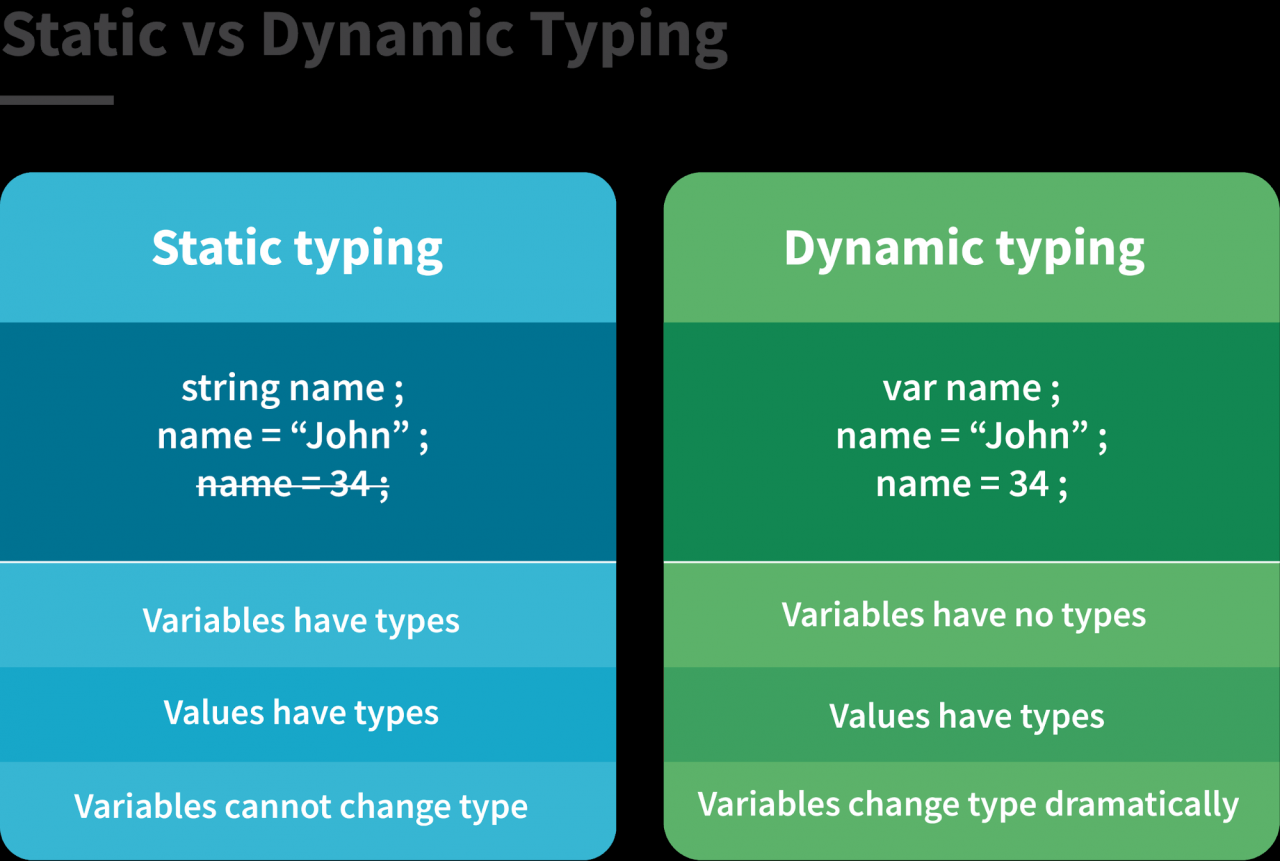``````var a = 23;
var a = "Hello World!";``````

### 6. JavaScript 中的 NaN 属性是什么？

NaN 属性表示“非数字”值。它表示一个不是合法数字的值。 NaN 的typeof将返回一个Number

**注意- isNaN() 函数将给定值转换为数字类型，然后等于 NaN。

``````isNaN("Hello")  // Returns true
isNaN(345)   // Returns false
isNaN('1')  // Returns false, since '1' is converted to Number type which results in 0 ( a number)
isNaN(true) // Returns false, since true converted to Number type results in 1 ( a number)
isNaN(false) // Returns false
isNaN(undefined) // Returns true``````

### 7.解释值传递和引用传递。

Javascript面试题解析：在 JavaScript 中，原始数据类型通过值传递，非原始数据类型通过引用传递。

``var x = 2;``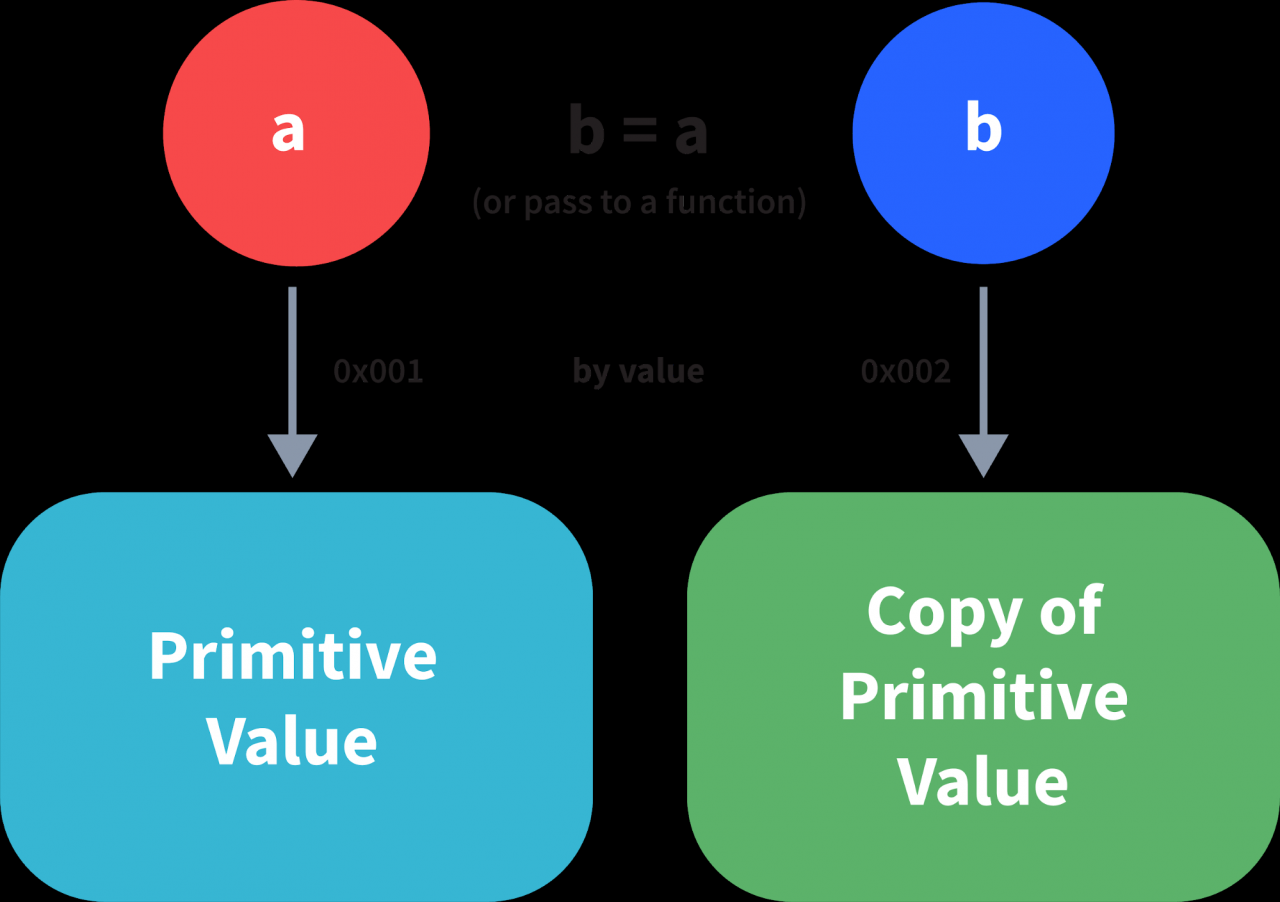``````var y = 234;
var z = y;``````

``````var y = #8454; // y pointing to address of the value 234

var z = y;

var z = #5411; // z pointing to a completely new address of the value 234

// Changing the value of y
y = 23;
console.log(z);  // Returns 234, since z points to a new address in the memory so changes in y will not effect z``````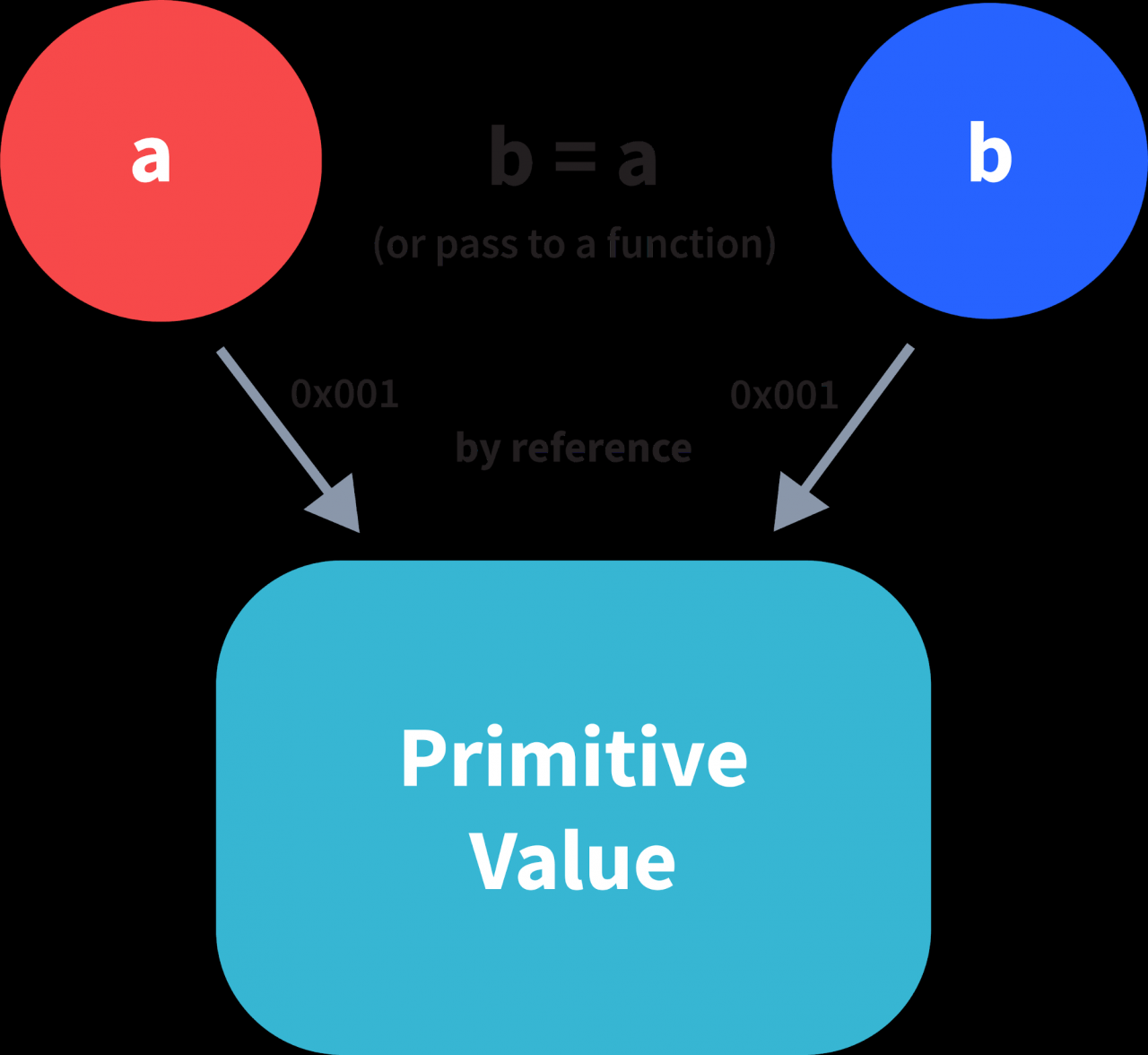``````var obj = { name: "Vivek", surname: "Bisht" };

var obj2 = obj;``````

``````var obj = #8711;  // obj pointing to address of { name: "Vivek", surname: "Bisht" }

var obj2 = obj;

var obj2 = #8711; // obj2 pointing to the same address

// changing the value of obj1

obj1.name = "Akki";

console.log(obj2);

// Returns {name:"Akki", surname:"Bisht"} since both the variables are pointing to the same address.``````

### 8. JavaScript 中的立即调用函数是什么？

IIFE 的语法：

``````(function(){
// Do something;
})();``````

``````(function (){
//Do something;
})``````

``````function() {
//Do something;
}
// Compiler gives an error since the syntax of declaring a function is wrong in the code above``````

``````(function (){
//Do something;
})();``````

``````(function (){
// Do something;
})

// Returns the function declaration``````

### 9. 用javascript解释高阶函数。

``````function higherOrder(fn) {
fn();
}

higherOrder(function() { console.log("Hello world") }); ``````
``````function higherOrder2() {
return function() {
return "Do something";
}
}

var x = higherOrder2();
x()   // Returns "Do something"``````

### 10.解释“this”关键字。

“this”关键字指的是函数是其属性的对象。

“this”关键字的值将始终取决于调用该函数的对象。

``````function doSomething() {
console.log(this);
}

doSomething();``````

#### “this”关键字指的是函数是其属性的对象。

``````var obj = {
name:  "vivek",
getName: function(){
console.log(this.name);
}
}

obj.getName();``````

`````` var obj = {
name:  "vivek",
getName: function(){
console.log(this.name);
}

}

var getName = obj.getName;

var obj2 = {name:"akshay", getName };
obj2.getName();``````

``````var obj1 = {
}
}

var obj2 = {name:"akshay"};

### 11. 解释 call()、apply() 和 bind() 方法。

call()

``````function sayHello(){
return "Hello " + this.name;
}

var obj = {name: "Sandy"};

sayHello.call(obj);

// Returns "Hello Sandy"
``````

call() 方法允许一个对象使用另一个对象的方法（函数）。

``````var person = {
age: 23,
getAge: function(){
return this.age;
}
}

var person2 = {age:  54};
person.getAge.call(person2);

// Returns 54  ``````

call() 接受参数：

``````function saySomething(message){
return this.name + " is " + message;
}

var person4 = {name:  "John"};

saySomething.call(person4, "awesome");
// Returns "John is awesome"    ``````

apply()

apply 方法类似于 call() 方法。唯一的区别是，

call() 方法单独接受参数，而 apply() 方法将参数作为数组。

``````function saySomething(message){
return this.name + " is " + message;
}

var person4 = {name:  "John"};

saySomething.apply(person4, ["awesome"]);``````

bind()

``````var bikeDetails = {
displayDetails: function(registrationNumber,brandName){
return this.name+ " , "+ "bike details: "+ registrationNumber + " , " + brandName;
}
}

var person1 = {name:  "Vivek"};

var detailsOfPerson1 = bikeDetails.displayDetails.bind(person1, "TS0122", "Bullet");

// Binds the displayDetails function to the person1 object

detailsOfPerson1();
// Returns Vivek, bike details: TS0452, Thunderbird``````

### 12. Javascript常见面试题有哪些：JavaScript 中的柯里化是什么？

``````function add (a) {
return function(b){
return a + b;
}
}

``````function multiply(a,b){
return a*b;
}

function currying(fn){
return function(a){
return function(b){
return fn(a,b);
}
}
}

var curriedMultiply = currying(multiply);

multiply(4, 3); // Returns 12

curriedMultiply(4)(3); // Also returns 12``````

### 13. 用javascript解释作用域和作用域链。

JS 中的作用域，决定了代码中各个部分的变量和函数的可访问性。

JS 中的作用域分为三种：

• 全局范围
• 局部或函数作用域
• 块作用域

``````var globalVariable = "Hello world";

function sendMessage(){
return globalVariable; // can access globalVariable since it's written in global space
}

function sendMessage2(){
return sendMessage(); // Can access sendMessage function since it's written in global space
}

sendMessage2();  // Returns “Hello world”``````

``````function awesomeFunction(){
var a = 2;

var multiplyBy2 = function(){
console.log(a*2); // Can access variable "a" since a and multiplyBy2 both are written inside the same function
}
}
console.log(a); // Throws reference error since a is written in local scope and cannot be accessed outside

multiplyBy2(); // Throws reference error since multiplyBy2 is written in local scope``````

``````{
let x = 45;
}

console.log(x); // Gives reference error since x cannot be accessed outside of the block

for(let i=0; i<2; i++){
// do something
}

console.log(i); // Gives reference error since i cannot be accessed outside of the for loop block``````

Scope Chain

JavaScript 引擎也使用 Scope 来查找变量。

``````var y = 24;

function favFunction(){
var x = 667;
var anotherFavFunction = function(){
console.log(x); // Does not find x inside anotherFavFunction, so looks for variable inside favFunction, outputs 667
}

var yetAnotherFavFunction = function(){
console.log(y); // Does not find y inside yetAnotherFavFunction, so looks for variable inside favFunction and does not find it, so looks for variable in global scope, finds it and outputs 24
}

anotherFavFunction();
yetAnotherFavFunction();
}

favFunction();
``````

### 14. 解释 JavaScript 中的闭包。

Javascript面试题解析：闭包是函数记住在其外部作用域中声明的变量和函数的能力。

``````var Person = function(pName){
var name = pName;

this.getName = function(){
return name;
}
}

var person = new Person("Neelesh");
console.log(person.getName());``````

``````function randomFunc(){
var obj1 = {name:"Vivian", age:45};

return function(){
console.log(obj1.name + " is "+ "awesome"); // Has access to obj1 even when the randomFunc function is executed

}
}

var initialiseClosure = randomFunc(); // Returns a function

initialiseClosure(); ``````

``var initialiseClosure = randomFunc();``

`` initialiseClosure(); ``

``console.log(obj1.name + " is "+ "awesome");``

### 15. 什么是对象原型？

Date 对象从 Date 原型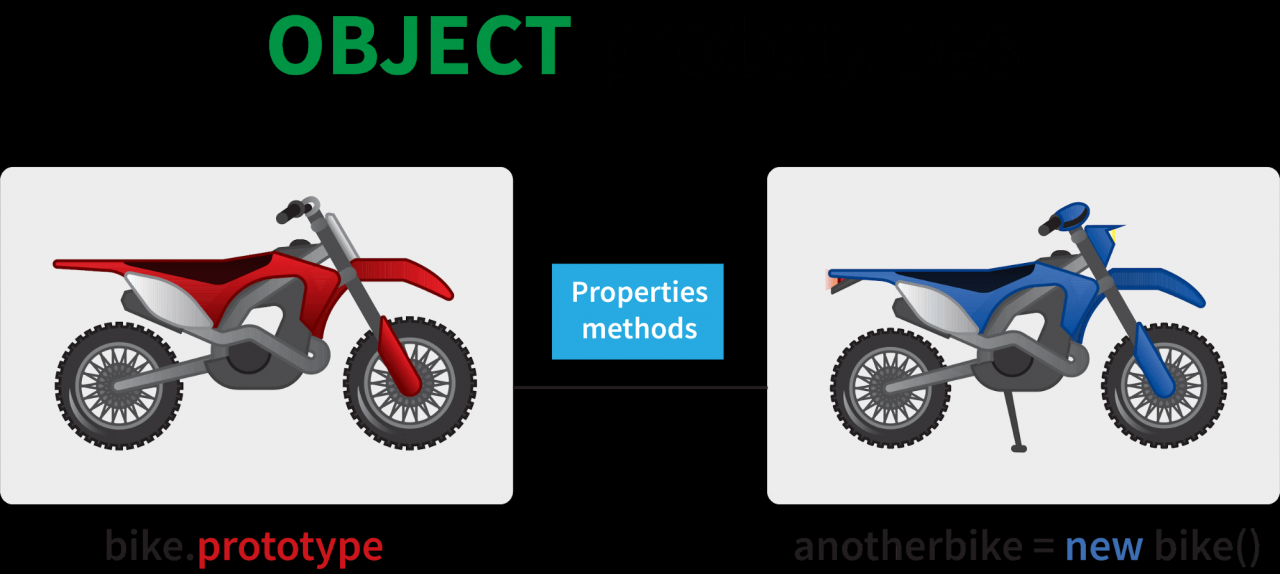``````var arr = [];
arr.push(2);

console.log(arr); // Outputs ``````

javascript 引擎发现当前数组对象上不存在 push 方法，因此在 Array 原型中查找方法 push 并找到该方法。

### 16. 什么是回调？

``````function divideByHalf(sum){
console.log(Math.floor(sum / 2));
}

function multiplyBy2(sum){
console.log(sum * 2);
}

function operationOnSum(num1,num2,operation){
var sum = num1 + num2;
operation(sum);
}

operationOnSum(3, 3, divideByHalf); // Outputs 3

operationOnSum(5, 5, multiplyBy2); // Outputs 20``````

operationOnSum 函数接受 3 个参数，第一个数字、第二个数字以及要对其总和执行的操作（回调）。

### 17.Javascript面试题和答案合集：什么是Memoization？

Memoization 是一种缓存形式，其中函数的返回值基于其参数进行缓存。如果该函数的参数未更改，则返回该函数的缓存版本。

**注意- Memoization 用于昂贵的函数调用，但在以下示例中，我们正在考虑使用一个简单的函数来更好地理解 memoization 的概念。

``````function addTo256(num){
return num + 256;
}

addTo256(20); // Returns 276
addTo256(40); // Returns 296
addTo256(20); // Returns 276``````

``````function memoizedAddTo256(){
var cache = {};

return function(num){
if(num in cache){
console.log("cached value");
return cache[num]

}
else{
cache[num] = num + 256;
return cache[num];
}
}
}

var memoizedFunc = memoizedAddTo256();

memoizedFunc(20); // Normal return
memoizedFunc(20); // Cached return``````

**注意- 尽管使用记忆化可以节省时间，但由于我们存储了所有计算结果，因此会导致更大的内存消耗。

### 18. 什么是编程语言中的递归？

``````function add(number) {
if (number <= 0) {
return 0;
} else {
return number + add(number - 1);
}
}

3 + 2 + add(1)
3 + 2 + 1 + add(0)
3 + 2 + 1 + 0 = 6  ``````

``````function computeSum(arr){
if(arr.length === 1){
return arr;
}
else{
return arr.pop() + computeSum(arr);
}
}

computeSum([7, 8, 9, 99]); // Returns 123``````

### 19. javascript中构造函数有什么用？

#### **注意- 构造函数的名称应始终以 Pascal Notation 书写：每个单词都应以大写字母开头。

``````function Person(name,age,gender){
this.name = name;
this.age = age;
this.gender = gender;
}

var person1 = new Person("Vivek", 76, "male");
console.log(person1);

var person2 = new Person("Courtney", 34, "female");
console.log(person2);``````

``var person3 = new Person("Lilly", 17, "female");``

### 20.Javascript常见面试题有哪些：什么是DOM？

DOM 代表文档对象模型。

DOM 是 HTML 和 XML 文档的编程接口。

HTML 代码如何转换为 DOM 的示例：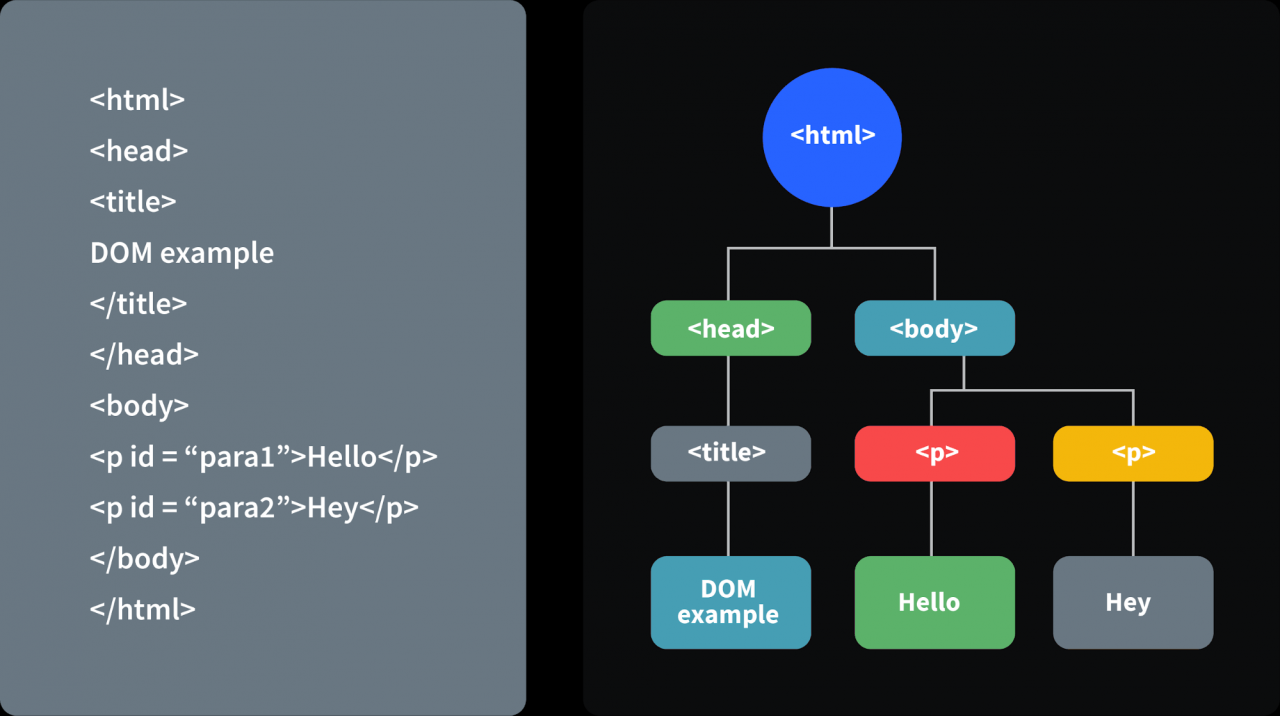# 高级 Javascript 面试问题：

### 21. 什么是箭头函数？

``````// 传统函数表达式
var add =  function (a,b){
return a + b;
}

// 箭头函数表达式
var arrowAdd = (a,b) => a + b;``````

``````// 传统函数表达式
var multiplyBy2 =  function (num){
return num *  2 ;
}
// 箭头函数表达式
var arrowMultiplyBy2 = num => num *  2 ;``````

``````var obj1 = {
valueOfThis: function(){
return this;
}
}
var obj2 = {
valueOfThis: ()=>{
return this;
}
}

obj1.valueOfThis(); // Will return the object obj1
obj2.valueOfThis(); // Will return window/global object``````

### 22. Javascript常见面试题有哪些：使用 var、let 和 const 声明变量的区别。

``````var variable1 = 23;

let variable2 = 89;

function catchValues(){
console.log(variable1);
console.log(variable2);

// Both the variables can be accessed anywhere since they are declared in the global scope
}

window.variable1; // Returns the value 23

window.variable2; // Returns undefined    ``````

var 与 let 在函数范围内

``````function varVsLetFunction(){
let awesomeCar1 = "Audi";
var awesomeCar2 = "Mercedes";
}

console.log(awesomeCar1); // Throws an error
console.log(awesomeCar2); // Throws an error``````

``````{
var variable3 = [1, 2, 3, 4];
}

console.log(variable3); // Outputs [1,2,3,4]

{
let variable4 = [6, 55, -1, 2];
}

console.log(variable4); // Throws error

for(let i = 0; i < 2; i++){
//Do something
}

console.log(i); // Throws error

for(var j = 0; j < 2; i++){
// Do something
}

console.log(j) // Outputs 2``````

let关键字声明的变量不能从块外部访问。

const 关键字

``````const x = {name:"Vivek"};

x = {address: "India"}; // Throws an error

x.name = "Nikhil"; // No error is thrown

const y = 23;

y = 44; // Throws an error``````

rest参数 ( … )

``````function extractingArgs(...args){
return args;
}

// extractingArgs(8,9,1); // Returns 9

let sumOfArgs = 0;
let i = 0;
while(i < args.length){
sumOfArgs += args[i];
i++;
}
return sumOfArgs;
}

addAllArgs(6, 5, 7, 99); // Returns 117
addAllArgs(1, 3, 4); // Returns 8``````

**注意- Rest 参数应始终用于函数的最后一个参数：

``````// Incorrect way to use rest parameter
function randomFunc(a,...args,c){
//Do something
}

// Correct way to use rest parameter
function randomFunc2(a,b,...args){
//Do something
}``````

``````function addFourNumbers(num1,num2,num3,num4){
return num1 + num2 + num3 + num4;
}

let fourNumbers = [5, 6, 7, 8];

// Spreads [5,6,7,8] as 5,6,7,8

let array1 = [3, 4, 5, 6];
let clonedArray1 = [...array1];
// Spreads the array into 3,4,5,6
console.log(clonedArray1); // Outputs [3,4,5,6]

let obj1 = {x:'Hello', y:'Bye'};
let clonedObj1 = {...obj1}; // Spreads and clones obj1
console.log(obj1);

let obj2 = {z:'Yes', a:'No'};
let mergedObj = {...obj1, ...obj2}; // Spreads both the objects and merges it
console.log(mergedObj);
// Outputs {x:'Hello', y:'Bye',z:'Yes',a:'No'};``````

***注意- 其余参数和扩展运算符之间的主要区别：

• Rest参数用于接受可变数量的参数并转换为数组，而扩展运算符则采用数组或对象并将其扩展
• Rest 参数用于函数声明，而扩展运算符用于函数调用。

### 24. javascript中promise有什么用？

Javascript面试题解析：Promise 用于处理 JavaScript 中的异步操作。

Promise 对象有四种状态 -

• Pending  - 承诺的初始状态。这个状态代表promise既没有被履行也没有被拒绝，处于pending状态。
• Fulfilled - 此状态表示承诺已完成，意味着异步操作已完成。
• Rejected - 此状态表示由于某种原因承诺已被拒绝，这意味着异步操作已失败。
• Settled  - 此状态表示承诺已被拒绝或已完成。

Promise 是使用Promise构造函数创建的，该构造函数接受一个带有两个参数的回调函数，分别是resolverejectresolve是一个将在异步操作成功完成后调用的函数。 当异步操作失败或发生某些错误时，

reject 是一个将被调用的函数。 Promise示例：

Promise 用于处理服务器请求等异步操作，为了便于理解，我们使用一个操作来计算三个元素的总和。

``````function sumOfThreeElements(...elements){
return new Promise((resolve,reject)=>{
if(elements.length > 3 ){
reject("Only three elements or less are allowed");
}
else{
let sum = 0;
let i = 0;
while(i < elements.length){
sum += elements[i];
i++;
}
resolve("Sum has been calculated: "+sum);
}
})
}``````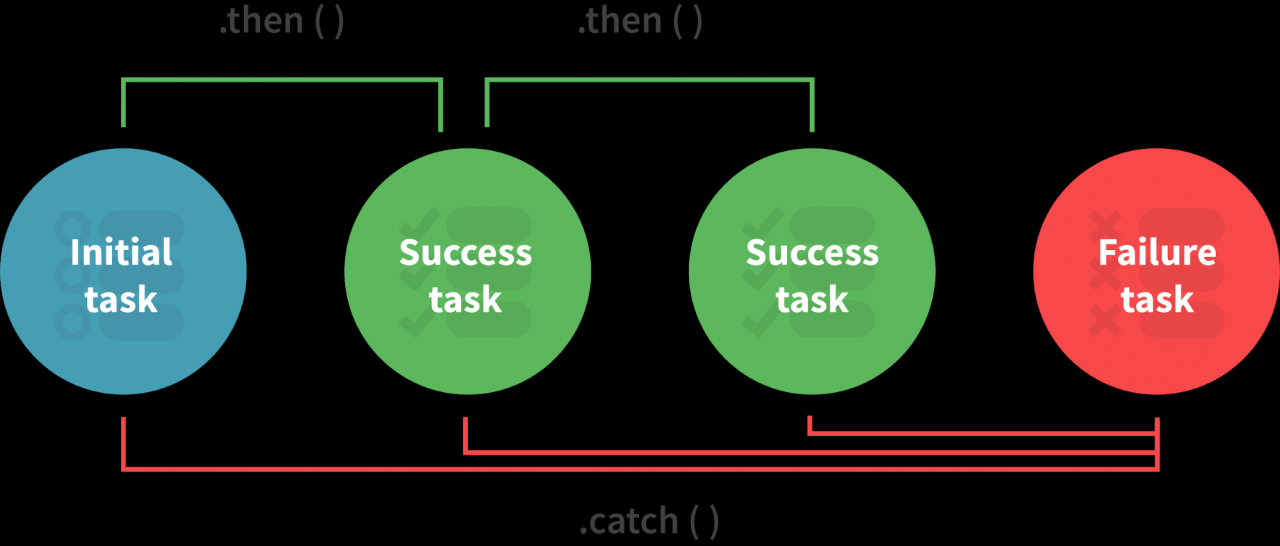then()方法用于在承诺完成时访问结果。 当承诺被拒绝时，

catch()方法用于访问结果/错误。

``````sumOfThreeElements(4, 5, 6)
.then(result=> console.log(result))
.catch(error=> console.log(error));
// In the code above, the promise is fulfilled so the then() method gets executed

sumOfThreeElements(7, 0, 33, 41)
.then(result => console.log(result))
.catch(error=> console.log(error));
// In the code above, the promise is rejected hence the catch() method gets executed``````

### 25.javascript中的类是什么？

``````// Before ES6 version, using constructor functions
this.name = name;
this.rollNumber = rollNumber;
this.section = section;
}

// Way to add methods to a constructor function
Student.prototype.getDetails = function(){
return 'Name: \${this.name}, Roll no: \${this.rollNumber}, Grade: \${this.grade}, Section:\${this.section}';
}

let student1 = new Student("Vivek", 354, "6th", "A");
student1.getDetails();
// Returns Name: Vivek, Roll no:354, Grade: 6th, Section:A

// ES6 version classes
class Student{
this.name = name;
this.rollNumber = rollNumber;
this.section = section;
}

// Methods can be directly added inside the class
getDetails(){
return 'Name: \${this.name}, Roll no: \${this.rollNumber}, Grade:\${this.grade}, Section:\${this.section}';
}
}

let student2 = new Student("Garry", 673, "7th", "C");
student2.getDetails();
// Returns Name: Garry, Roll no:673, Grade: 7th, Section:C``````

• 与函数不同，类不会被提升。类在声明之前不能使用。
• 一个类可以通过使用 extend 关键字从其他类继承属性和方法。
• 类中的所有语法都必须遵循 javascript 的严格模式（'use strict'）。如果不遵循严格模式规则，则会抛出错误。

### 26. 什么是生成器函数？

``````function* genFunc(){
// Perform operation
}``````

``````function normalFunc(){
return 22;
console.log(2); // This line of code does not get executed
}``````

``````function* genFunc(){
yield 3;
yield 4;
}
genFunc(); // Returns Object [Generator] {}
``````

``genFunc().next(); // Returns {value: 3, done:false}``

Value 属性表示产生的值。
Done 属性告诉我们函数代码是否完成。（如果完成则返回 true）

``````function* iteratorFunc() {
let count = 0;
for (let i = 0; i < 2; i++) {
count++;
yield i;
}
return count;
}

let iterator = iteratorFunc();
console.log(iterator.next()); // {value:0,done:false}
console.log(iterator.next()); // {value:1,done:false}
console.log(iterator.next()); // {value:2,done:true}``````

### 27. 用javascript解释WeakSet。

• Weakset 只包含对象，不包含其他类型。
• 弱集内的对象被弱引用。这意味着，如果弱集内的对象没有引用，它将被垃圾收集。
• 与 Set 不同，WeakSet 只有三个方法，add()delete()has()
``````const newSet = new Set([4, 5, 6, 7]);
console.log(newSet);// Outputs Set {4,5,6,7}

const newSet2 = new WeakSet([3, 4, 5]); //Throws an error

let obj1 = {message:"Hello world"};
const newSet3 = new WeakSet([obj1]);
console.log(newSet3.has(obj1)); // true``````

### 28. 用javascript解释WeakMap。

Javascript面试题解析：在 JavaScript 中，Map 用于存储键值对。键值对可以是原始类型和非原始类型。

WeakMap 与 Map 类似，但主要区别在于：

• weakmap 中的键和值应该始终是一个对象。
• 如果没有对该对象的引用，则该对象将被垃圾回收。
``````const map1 = new Map();
map1.set('Value', 1);

const map2 = new WeakMap();
map2.set('Value', 2.3); // Throws an error

let obj = {name:"Vivek"};
const map3 = new WeakMap();
map3.set(obj, {age:23});``````

### 29. 什么是对象解构？

ES6 版本之前：

``````const classDetails = {
strength: 78,
benches: 39,
blackBoard:1
}

const classStrength = classDetails.strength;
const classBenches = classDetails.benches;
const classBlackBoard = classDetails.blackBoard;``````

``````const classDetails = {
strength: 78,
benches: 39,
blackBoard:1
}

const {strength:classStrength, benches:classBenches,blackBoard:classBlackBoard} = classDetails;

console.log(classStrength); // Outputs 78
console.log(classBenches); // Outputs 39
console.log(classBlackBoard); // Outputs 1``````

``````const {strength:strength} = classDetails;
// The above line of code can be written as:
const {strength} = classDetails;``````

#### 数组解构：

ES6版本之前：

``````const arr = [1, 2, 3, 4];
const first = arr;
const second = arr;
const third = arr;
const fourth = arr;``````

``````const arr = [1, 2, 3, 4];
const [first,second,third,fourth] = arr;

console.log(first); // Outputs 1
console.log(second); // Outputs 2
console.log(third); // Outputs 3
console.log(fourth); // Outputs 4``````

### 30. 什么是时间死区？

Javascript面试题和答案合集：时间死区是使用letconst关键字声明的变量时发生的行为。

``````x = 23; // Gives reference error

let x;

function anotherRandomFunc(){
message = "Hello"; // Throws a reference error

let message;
}
anotherRandomFunc();``````

## 编码问题：

### 31. 猜以下代码的输出：

``````// Code 1:

function func1(){
setTimeout(()=>{
console.log(x);
console.log(y);
},3000);

var x = 2;
let y = 12;
}

func1();

// Code 2:

function func2(){
for(var i = 0; i < 3; i++){
setTimeout(()=> console.log(i),2000);
}

}

func2();

// Code 3:

(function(){
setTimeout(()=> console.log(1),2000);
console.log(2);
setTimeout(()=> console.log(3),0);
console.log(4);
})();
``````

``````2
4
3
1 // After two seconds``````

### 32.猜以下代码的输出：

``````// Code 1:

let x= {}, y = {name:"Ronny"},z = {name:"John"};

x[y] = {name:"Vivek"};
x[z] = {name:"Akki"};

console.log(x[y]);

// Code 2:

function runFunc(){
console.log("1" + 1);
console.log("A" - 1);
console.log(2 + "-2" + "2");
console.log("Hello" - "World" + 78);
console.log("Hello"+ "78");
}

runFunc();

// Code 3:

let a = 0;
let b = false;
console.log((a == b));
console.log((a === b));``````

x[y] = {name:”Vivek”}和写x['object Object'] = {name:”Vivek”} 一样

x[y] 和 x[z] 都引用相同的属性。

``````11
Nan
2-22
NaN
Hello78``````

``````true
false``````

### 33.猜猜下面代码的输出：

``````var x = 23;

(function(){
var x = 43;
(function random(){
x++;
console.log(x);
var x = 21;
})();
})();``````

random() 函数具有函数作用域，因为 x 在函数作用域中被声明和提升。

``````function random(){
var x; // x is hoisted
x++; // x is not a number since it is not initialized yet
console.log(x); // Outputs NaN
x = 21; // Initialization of x
}``````

### 34. 猜猜以下代码的输出：

``````// Code 1

let hero = {
powerLevel: 99,
getPower(){
return this.powerLevel;
}
}

let getPower = hero.getPower;

let hero2 = {powerLevel:42};
console.log(getPower());
console.log(getPower.apply(hero2));

// Code 2

const a = function(){
console.log(this);

const b = {
func1: function(){
console.log(this);
}
}

const c = {
func2: ()=>{
console.log(this);
}
}

b.func1();
c.func2();
}

a();

// Code 3

const b = {
name:"Vivek",
f: function(){
var self = this;
console.log(this.name);
(function(){
console.log(this.name);
console.log(self.name);
})();
}
}

b.f();``````

``````undefined
42  ``````

``window.getPower() = getPower();``

``````global/window object
object "b"
global/window object``````

``````"Vivek"
undefined
"Vivek" ``````

### 35. 猜猜以下代码的输出：

**注意 - 代码 2 和代码 3 要求你修改代码，而不是猜测输出。

``````// Code 1

(function(a){
return (function(){
console.log(a);
a = 23;
})()
})(45);

// Code 2

// Each time bigFunc is called, an array of size 700 is being created,
// Modify the code so that we don't create the same array again and again

function bigFunc(element){
let newArray = new Array(700).fill('♥');
return newArray[element];
}

console.log(bigFunc(599)); // Array is created
console.log(bigFunc(670)); // Array is created again

// Code 3

// The following code outputs 2 and 2 after waiting for one second
// Modify the code to output 0 and 1 after one second.

function randomFunc(){
for(var i = 0; i < 2; i++){
setTimeout(()=> console.log(i),1000);
}
}

randomFunc();``````

``````function bigFunc(){
let newArray = new Array(700).fill('♥');
return (element) => newArray[element];
}

let getElement = bigFunc(); // Array is created only once
getElement(599);
getElement(670);  ``````

``````function randomFunc(){
for(let i = 0; i < 2; i++){
setTimeout(()=> console.log(i),1000);
}
}

randomFunc(); ``````

``````function randomFunc(){
for(var i = 0; i < 2; i++){
(function(i){
setTimeout(()=>console.log(i),1000);
})(i);
}
}

randomFunc();  ``````

### 36. 编写一个对排序数组执行二分查找的函数。

``````function binarySearch(arr,value,startPos,endPos){
if(startPos > endPos) return -1;

let middleIndex = Math.floor(startPos+endPos)/2;

if(arr[middleIndex] === value) return middleIndex;

elsif(arr[middleIndex > value]){
return binarySearch(arr,value,startPos,middleIndex-1);
}
else{
return binarySearch(arr,value,middleIndex+1,endPos);
}
}   ``````

### 37.实现返回使用更新的数组的函数[R整数数组上向右旋转一个。

[2,3,4,5,7]

``````function rotateRight(arr,rotations){
if(rotations == 0) return arr;
for(let i = 0; i < rotations;i++){
let element = arr.pop();
arr.unshift(element);
}

return arr;
}

rotateRight([2, 3, 4, 5, 7], 3); // Return [4,5,7,2,3]
rotateRight([44, 1, 22, 111], 5); // Returns [111,44,1,22]   ``````